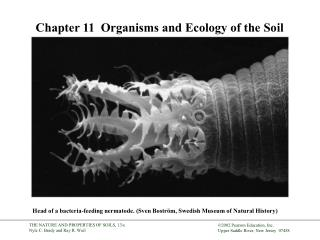DownloadDownload PresentationChapter 11 Organisms and Ecology of the Soil

# Chapter 11 Organisms and Ecology of the Soil

Download Presentation## Chapter 11 Organisms and Ecology of the Soil

- - - - - - - - - - - - - - - - - - - - - - - - - - - E N D - - - - - - - - - - - - - - - - - - - - - - - - - - -
##### Presentation Transcript

1. Chapter 11 Organisms and Ecology of the Soil Head of a bacteria-feeding nermatode. (Sven Boström, Swedish Museum of Natural History)

2. Figure 11.1

3. Figure 11.2

4. Figure 11.3

5. Figure 11.4

6. Figure 11.5

7. Figure 11.6

8. Figure 11.9

9. Figure 11.10

10. Figure 11.12

11. Figure 11.13 (a) (b)

12. Figure 11.14

13. Figure 11.17

14. Figure 11.18

15. Figure 11.19

16. Figure 11.20

17. Figure 11.21 (a) (b)

18. Figure 11.22

19. Figure 11.23

20. Figure 11.25

21. Figure 11.31

22. Figure 11.34